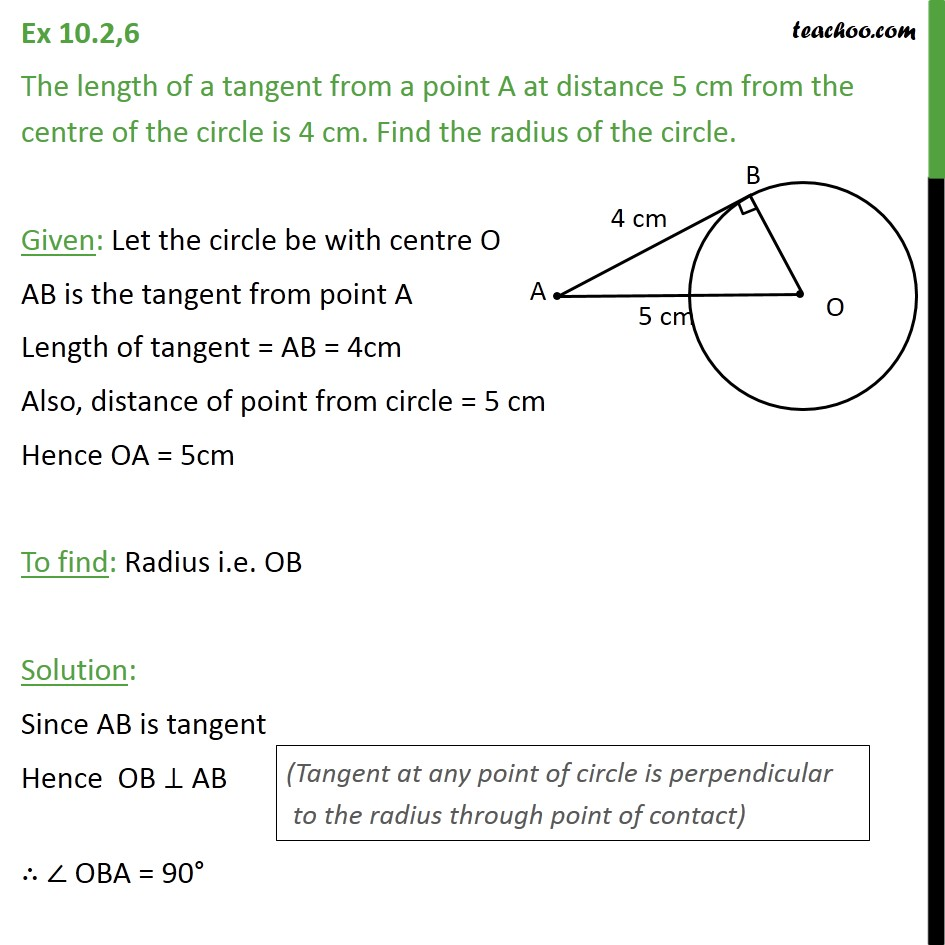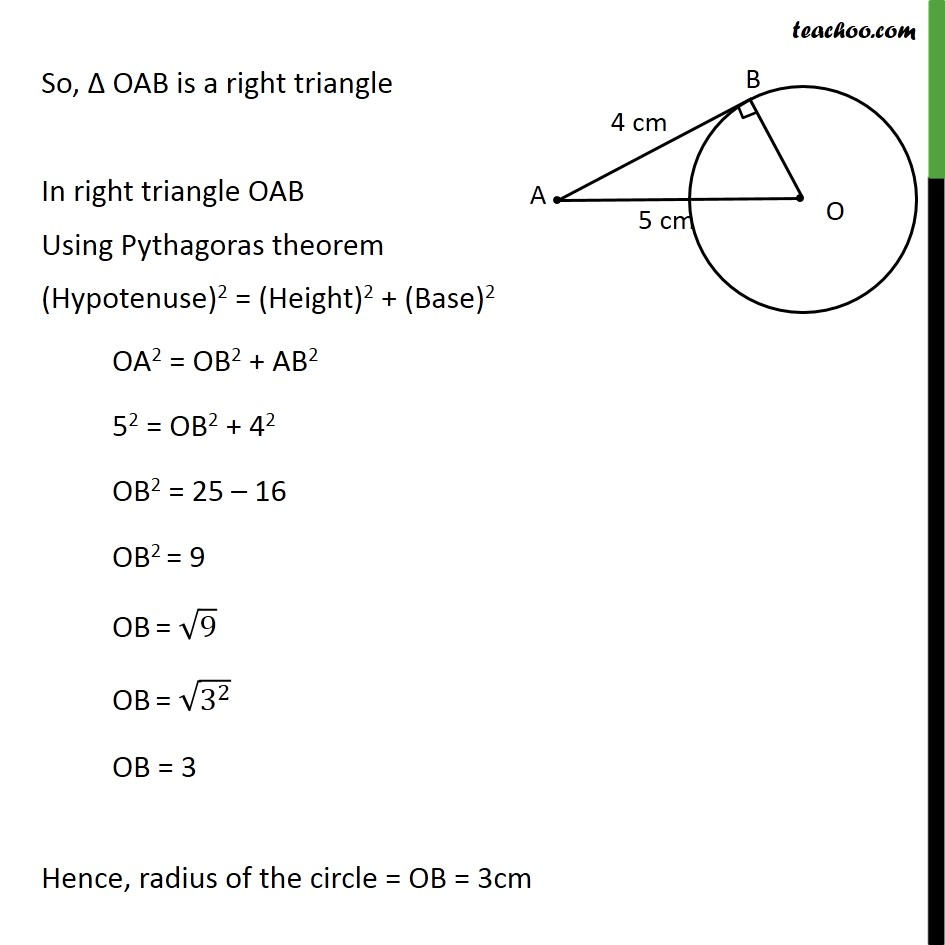Theorem 10.1: Tangent perpendicular to radius (numerical type)Learn in your speed, with individual attention - Teachoo Maths 1-on-1 Class

### Transcript

Ex 10.2,6 The length of a tangent from a point A at distance 5 cm from the centre of the circle is 4 cm. Find the radius of the circle. Given: Let the circle be with centre O AB is the tangent from point A Length of tangent = AB = 4cm Also, distance of point from circle = 5 cm Hence OA = 5cm To find: Radius i.e. OB Solution: Since AB is tangent Hence OB AB OBA = 90 So, OAB is a right triangle In right triangle OAB Using Pythagoras theorem (Hypotenuse)2 = (Height)2 + (Base)2 OA2 = OB2 + AB2 52 = OB2 + 42 OB2 = 25 16 OB2 = 9 OB = 9 OB = (3^2 ) OB = 3 Hence, radius of the circle = OB = 3cm